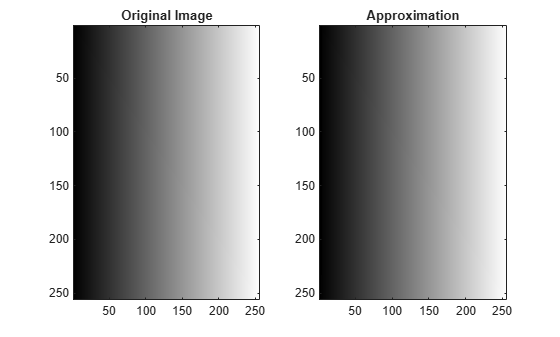# measerr

Quality metrics of signal or image approximation

## Syntax

``````[PSNR,MSE,MAXERR,L2RAT] = measerr(X,XAPP)``````
``[PSNR,MSE,MAXERR,L2RAT] = measerr(X,XAPP,BPS)``

## Description

example

``````[PSNR,MSE,MAXERR,L2RAT] = measerr(X,XAPP)``` returns the peak signal-to-noise ratio, `PSNR`, mean square error, `MSE`, maximum squared error, `MAXERR`, and ratio of squared norms, `L2RAT`, for an input signal or image, `X`, and its approximation, `XAPP`.```

example

````[PSNR,MSE,MAXERR,L2RAT] = measerr(X,XAPP,BPS)` uses the bits per sample, `BPS`, to determine the peak signal-to-noise ratio.```

## Examples

collapse all

Approximate an RGB image and compute the quality metrics.

Load an RGB image. Return the image dimensions and minimum and maximum values.

```X = imread('africasculpt.jpg'); size(X)```
```ans = 1×3 512 512 3 ```
`[min(X(:)) max(X(:))]`
```ans = 1x2 uint8 row vector 0 236 ```

Define the image approximation by setting equal to 1 all RGB values less than or equal to 100.

```Xapp = X; Xapp(X<=100) = 1;```

Display the image and its approximation.

```subplot(1,2,1) image(X) title('Original Image') subplot(1,2,2) image(Xapp) title('Approximation')```Compute the quality metrics of the image approximation.

`[psnr,mse,maxerr,L2rat] = measerr(X,Xapp)`
```psnr = 17.5287 ```
```mse = 1.1487e+03 ```
```maxerr = 99 ```
```L2rat = 0.9398 ```

Approximate a grayscale image and calculate approximation quality metrics.

Create a 256-by-256 grayscale image with intensities between $0$ and ${2}^{16}-1$.

```val = 0:2^16-1; X = reshape(val,256,256);```

There are 16 bits per sample. Define the image approximation by setting equal to 1 all grayscale values less than or equal to 1000. Display the image and its approximation.

```Xapp = X; Xapp(X<=1000) = 1; colormap(gray(2^16)) subplot(1,2,1) image(X) title('Original Image') subplot(1,2,2) image(Xapp) title('Approximation')```There are 16 bits per sample. Compute the quality metrics of the grayscale approximation.

```bps = 16; [psnr,mse,maxerr,L2rat] = measerr(X,Xapp)```
```psnr = 11.0733 ```
```mse = 5.0786e+03 ```
```maxerr = 999 ```
```L2rat = 1.0000 ```

## Input Arguments

collapse all

Input signal or image, specified as a real-valued array.

Approximation of signal or image `X`, specified as a real-valued array. `XAPP` is the same size as `X`.

Bits per sample of the input data, specified as a positive integer. The default value is `8`, so the maximum possible pixel value of an image (MAXI) is 255. More generally, when samples are represented using linear Pulse Code Modulation with B bits per sample, MAXI is 2B−1.

## Output Arguments

collapse all

Peak signal-to-noise ratio (PSNR) in decibels, returned as a positive real number. The PSNR is only meaningful for data encoded in terms of bits per sample or bits per pixel. For example, an image with 8 bits per pixel contains integers from 0 to 255.

Mean square error, returned as a positive real number. `MSE` is the squared norm of the difference between `X` and `XAPP` divided by the number of elements.

Maximum absolute squared deviation of the data `X` from the approximation `XAPP`, returned as a positive real number.

Energy ratio between the approximation `XAPP` and input data `X`, returned as a positive real number. `L2RAT` is the ratio of the squared norm of `XAPP` to `X`.

collapse all

### Peak Signal to Noise Ratio

The peak signal-to-noise ratio (PSNR) in decibels between a signal and its approximation is

`$20{\mathrm{log}}_{10}\left(\frac{{2}^{B}-1}{\sqrt{MSE}}\right)$`

where MSE represents the mean square error, and B represents the bits per sample.

### Mean Square Error

The mean square error (MSE) between a signal or image, X, and an approximation, Y, is

`$\frac{||X-Y|{|}^{2}}{N}$`

where N is the number of elements in the signal.

 Huynh-Thu, Q. and M. Ghanbari. "Scope of Validity of PSNR in Image/Video Quality Assessment." Electronics Letters. Vol. 44, Issue 13, 2008, pp. 800–801.

## SupportGet trial now